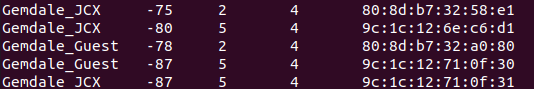# 原创 C++解析一些特殊符号tab、换行符号

2021-5-12 19:49 392 1 1 分类: MCU/ 嵌入式 文集: 嵌入式前言：int i, j ;
i = 0;
j = strlen(strIn) - 1;
while(strIn == ' ' || strIn == '\t')
++i;

while(strIn[j] == ' ' || strIn[j] == '\t')
--j;
strncpy(strOut, strIn + i , j - i + 1);

{
vector words;
if(msg.empty())
return words;
string::iterator temp_p = msg.begin();
string sepword;
bool bit_true = false;
while(temp_p != msg.end())
{
if(/* * temp_p == ' ' || */  * temp_p == '\t' || * temp_p == '\n')
{
if(bit_true)
{
words.push_back(sepword);
PP_INFO("seword :%s",sepword.c_str());
sepword.clear();
}
bit_true = false;
temp_p++;
continue;
}
else
{
bit_true = true;
sepword += *temp_p;
}
if(*temp_p ++ == '\0')
{
break;
}
// else
// {
//  PP_INFO("%d %d %c",msg.end(),*temp_p,*temp_p);
// }
}
// for(auto point : words)
// {
//  printf(".%s\n",point.c_str());
// }
//for(uint16_t i =0;i      //{
// printf("[]%s,%s\n",words.c_str(),words[i+1].c_str(),words[i+3].c_str());
//}
return words;
}

‧‧‧‧‧‧‧‧‧‧‧‧‧‧‧‧ END ‧‧‧‧‧‧‧‧‧‧‧‧‧‧‧‧

【3】CPU中的程序是怎么运行起来的 必读

## 文章评论（0条评论）良知犹存 2021-05-10 09:57
C语言0数组\柔性数组使用介绍良知犹存 2021-03-06 19:38良知犹存 2021-02-06 09:24良知犹存 2021-02-05 20:04良知犹存 2021-01-18 14:16
STM32通过rosserial接入ROS通讯开发

EE直播间

0
1
1
2
3
4
5
6
7
8
9
0